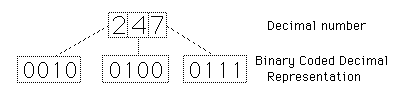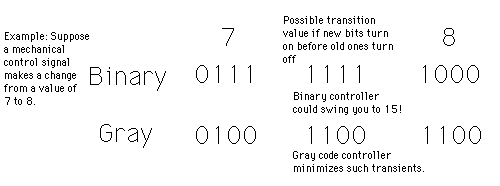# Binary Coded Decimal

One of the most widely used representations of numerical data is the binary coded decimal (BCD) form in which each integer of a decimal number is represented by a 4-bit binary number (see conversion table). It is particularly useful for the driving of display devices where a decimal output is desired. BCD usually refers to such coding in which the binary digits have their normal values, i.e., 8421. Sometimes it is written "8421 BCD" to clearly distinguish it from other binary codes such as the 4221 Code, but when BCD is used without qualification, the 8421 version is assumed.Number Systems
 Construction of a BCD Counter
Index

Electronics concepts

Digital Circuits

 HyperPhysics*****Electricity and magnetism R Nave
Go Back

# Excess-3 Code

In this code, a digit is represented by adding 3 to the number and then converting it to a 4-bit binary number. It can be used for the representation of multi-digit decimal numbers as can BCD. This code along with the 4221 code have some advantages for performing decimal arithmetic.

 Number Systems
Index

Electronics concepts

Digital Circuits

 HyperPhysics*****Electricity and magnetism R Nave
Go Back

# 4221 Code

There are several codes which use binary numbers to code for single digits in multi-digit decimal numbers. This code assigns the values 4,2,2,1 instead of 8,4,2,1 to the four binary digits. It can properly be called binary coded decimal, but that designation (BCD) is usually reserved for the 8,4,2,1 code. This code is sometimes written 4221 BCD. This code along with the excess-3 code have some advantages for performing decimal arithmetic.

 Number Systems
Index

Electronics concepts

Digital Circuits

 HyperPhysics*****Electricity and magnetism R Nave
Go Back

# Gray Code

A code designed to minimize errors during the transition between one code value and another, this code changes only one bit from one sequential value to another. It is used for shaft encoders and other applications where mechanical control using a digital code requires the minimizing of stresses which might occur if an erronius value was sent during a transition. The rule for generating the code is: begin with all zeros and change the least significant bit that will bring you to a new state.Number Systems
Index

Electronics concepts

Digital Circuits

 HyperPhysics*****Electricity and magnetism R Nave
Go Back